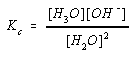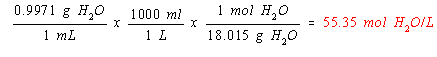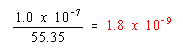Water, Acids, and Bases

Water, Acids, and Bases

The Acid-Base Chemistry of Water

The chemistry of aqueous solutions is dominated by the equilibrium between neutral water molecules and the ions they form.

2 H2O(l)H3O+(aq) + OH-(aq)

Strict application of the rules for writing equilibrium constant expressions to this reaction produces the following result.This is a legitimate equilibrium constant expression, but it fails to take into account the enormous difference between the concentrations of neutral H2O molecules and H3O+ and OH- ions at equilibrium.

Measurements of the ability of water to conduct an electric current suggest that pure water at 25oC contains 1.0 x 10-7 moles per liter of each of these ions.

[H3O+] = [OH-] = 1.0 x 10-7 M

At the same temperature, the concentration of neutral H2O molecules is 55.35 molar.The ratio of the concentration of the H+ (or OH-) ion to the concentration of the neutral H2O molecules is therefore 1.8 x 10-9.In other words, only about 2 parts per billion (ppb) of the water molecules dissociate into ions at room temperature.

The equilibrium concentration of H2O molecules is so much larger than the concentrations of the H3O+ and OH- ions that it is effectively constant. We therefore build the [H2O] term into the equilibrium constant for the reaction and thereby greatly simplify equilibrium calculations. We start by rearranging the equilibrium constant expression for the dissociation of water to give the following equation.

[H3O+][OH-] = Kc x [H2O]2

We then replace the term on the right side of this equation with a constant known as the waterdissociation equilibrium constant, Kw.

[H3O+][OH-] = Kw

In pure water, at 25C, the [H3O+] and [OH-] ion concentrations are 1.0 x 10-7 M. The value of Kw at 25C is therefore 1.0 x 10-14.

 [1.0 x 10-7][1.0 x 10-7] = 1.0 x 10-14 (at 25C)

Although Kw is defined in terms of the dissociation of water, this equilibrium constant expression is equally valid for solutions of acids and bases dissolved in water. Regardless of the source of the H3O+ and OH- ions in water, the product of the concentrations of these ions at equilibrium at 25C is always 1.0 x 10-14.Strong Acids and the H3O+ and OH- Ion Concentrations

Suppose we add enough strong acid to a beaker of water to raise the H3O+ ion concentration to 0.010 M. According to LeChatelier's principle, this should drive the equilibrium between water and its ions to the left, reducing the number of H3O+ and OH- ions in the solution.

2 H2O(l)H3O+(aq) + OH-(aq)

Because there are so many H3O+ ions in this solution, the change in the concentration of this ion is too small to notice. When the system returns to equilibrium, the H3O+ ion concentration is still about 0.010 M. Furthermore, when the reaction returns to equilibrium, the product of the H3O+ and OH- ion concentrations is once again equal to Kw.

[H3O+][OH-] = 1 x 10-14

The solution therefore comes back to equilibrium when the dissociation of water is so small that the OH- ion concentration is only 1.0 x 10-12 M.Adding an acid to water therefore has an effect on the concentration of both the H3O+ and OH- ions. Because it is a source of this ion, adding an acid to water increases the concentration of the H3O+ ion. Adding an acid to water, however, decreases the extent to which water dissociates. It therefore leads to a significant decrease in the concentration of the OH- ion.

As might be expected, the opposite effect is observed when a base is added to water. Because we are adding a base, the OH- ion concentration increases. Once the system returns to equilibrium, the product of the H3O+ and OH- ion concentrations is once again equal to Kw. The only way this can be achieved, of course, is by decreasing the concentration of the H3O+ ion.